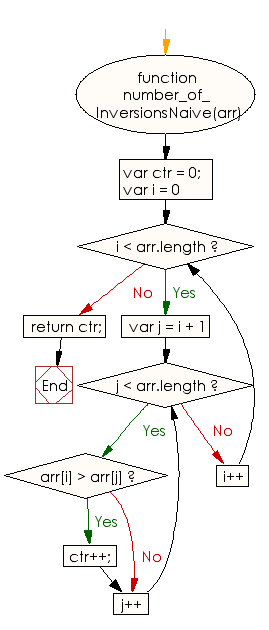# JavaScript: Find the number of inversions of a specified array of integers

## JavaScript Basic: Exercise-102 with Solution

Write a JavaScript program to find the number of inversions of a given array of integers.

Note: Two elements of the array a stored at positions i and j form an inversion if a[i] > a[j] and i < j.

Sample Solution:

HTML Code:

``````<!DOCTYPE html>
<html>
<meta charset="utf-8">
<meta name="viewport" content="width=device-width">
<title> Find the number of inversions of a specified array of integers</title>
<body>

</body>
</html>
```
```

JavaScript Code:

``````function number_of_InversionsNaive(arr) {
var ctr = 0;
for (var i = 0; i < arr.length; i++) {
for (var j = i + 1; j < arr.length; j++) {
if (arr[i] > arr[j])
ctr++;
}
}
return ctr;
}

console.log(number_of_InversionsNaive([0, 3, 2, 5, 9]));
console.log(number_of_InversionsNaive([1, 5, 4, 3]));
console.log(number_of_InversionsNaive([10, 30, 20, -10]));
``````

Sample Output:

```1
3
4
```

Flowchart:ES6 Version:

``````function number_of_InversionsNaive(arr) {
let ctr = 0;
for (let i = 0; i < arr.length; i++) {
for (let j = i + 1; j < arr.length; j++) {
if (arr[i] > arr[j])
ctr++;
}
}
return ctr;
}

console.log(number_of_InversionsNaive([0, 3, 2, 5, 9]));
console.log(number_of_InversionsNaive([1, 5, 4, 3]));
console.log(number_of_InversionsNaive([10, 30, 20, -10]));
``````

Live Demo:

See the Pen javascript-basic-exercise-102 by w3resource (@w3resource) on CodePen.

What is the difficulty level of this exercise?

Test your Programming skills with w3resource's quiz.

﻿

## JavaScript: Tips of the Day

Checks if a string is an anagram of another string (case-insensitive, ignores spaces, punctuation and special characters)

Example:

```const isAnagram = (str1, str2) => {
const normalize = str =>
str
.toLowerCase()
.replace(/[^a-z0-9]/gi, '')
.split('')
.sort()
.join('');
return normalize(str1) === normalize(str2);
};
console.log(isAnagram('iceman', 'cinema')); // true
```

Output:

```true
```